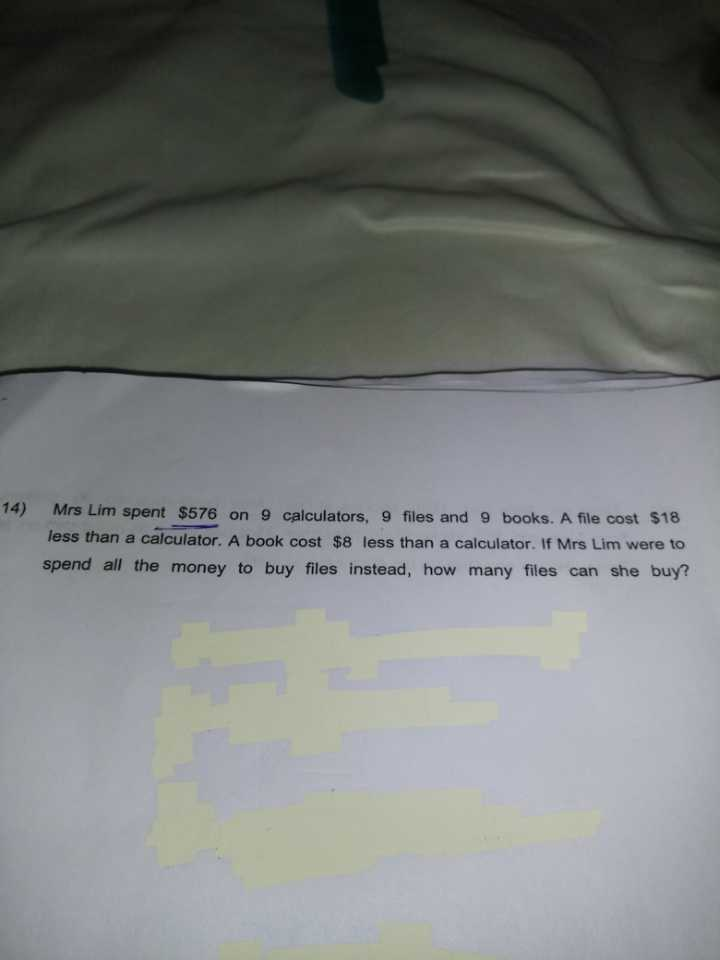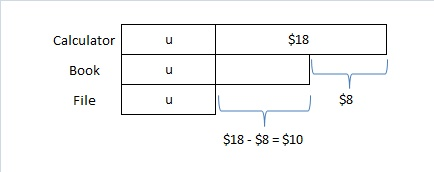QuestionCost

Calculator -> u + 18

Book -> u + 10

File -> u

Cost of 9C, 9B, 9F -> 9 × (u + 18 + u + 10 + u) = 27u + 252

27u + 252 = 576

27u = 324

u = 12

Each file cost \$12

If he were to spend \$576 all on files, number of files -> 576 ÷ 12 = 48